﻿ 稀疏化的因子分解机
«上一篇文章快速检索 高级检索

 智能系统学报2017, Vol. 12Issue (6): 816-822  DOI: 10.11992/tis.2017060300

### 引用本文GUO Shaocheng, CHEN Songcan. Sparsified factorization machine[J]. CAAI Transactions on Intelligent Systems, 2017, 12(6): 816-822. DOI: 10.11992/tis.201706030.### 文章历史

Sparsified factorization machine
GUO Shaocheng, CHEN SongcanCollege of Computer Science and Technology, Nanjing University of Aeronautics and Astronautics, Nanjing 210016, China
Abstract: Factorization machine (FM) is a recently proposed second-order linear model. One of its main advantages is that the interactions within it are factorized, making it suitable for data with high dimensionality and high sparsity. Though FM has been applied in recommender systems, it fails to consider the sparsity of variables explicitly, especially when the variable contains information on structural sparsity. Therefore, the process of feature selection should meet the following requirements: the linear terms and second-order terms that share the same feature should be included or excluded at the same time; when the feature is noise, both should be excluded, otherwise, both should be included. Based on the sparse structure described above, this paper proposes a sparse group lasso-based factorization machine (SGL-FM). By adding sparse group lasso to the loss function, SGL-FM not only achieves sparsity between groups but also within groups. From another point of view, sparsity within groups can be seen as a method of controlling the dimensionality of the factorization; therefore, SGL-FM chooses the best k automatically when faced with datasets with different properties. The experimental results show that by applying the proposed method, under conditions of excellent precision, a model with more sparsity than FM was obtained.
Key words: factorization machine    sparsity    sparse group lasso    feature selection    recommender systems

Group Lasso(GL) 是M.Yuan等基于Lasso提出的用于对组变量进行特征选择的方法，与Lasso不同的是，GL能同时选择或者不选组内的所有变量。首先根据先验知识将变量按照相关性划分为不同组，从聚类角度看就是将同类变量划分为同组，不同类变量划分为不同组。在FM中，将关于特征xi的线性项系数ωi和其在隐空间的特征表示向量vi分在同组中，这样，GL就能保证当xi是噪音时，ωivi同时不选，反之，则同时选择。虽然GL能实现这种结构稀疏，但是，对于选中的组，并不是所有特征都是有用的。因此，GL的使用有非常大限制，有必要继续选择组内重要的特征。

Simon等在GL的基础上提出了基于Sparse Group Lasso（SGL）的线性回归模型。与GL相同的是它们都对变量进行分组，与GL不同的是, SGL在GL的基础上，继续选择组内重要特征。因此，SGL能同时实现组间稀疏和组内稀疏, 而GL只实现了组间稀疏。SGL结合了Lasso和GL的优点，当待求变量存在结构稀疏信息时，仅使用Lasso会丢失结构信息，而仅使用GL又会导致求得冗余的解。基于上述事实，SGL既保留了GL的结构信息，又具有Lasso的高效特征选择的能力。

1 因子分解机 1.1 目标函数

FM的基本模型如下：

 $\hat y({\mathit{\boldsymbol{x}}}) = {\omega _0} + {\mathop \sum \limits_{i = 1}^p} {{\mathit{\boldsymbol{\omega }}}_i}{{\mathit{\boldsymbol{x}}}_i} +{\mathop \sum \limits_{i = 1}^p} {\mathop \sum \limits_{j = i + 1}^p} < {{\mathit{\boldsymbol{v}}}_i},{{\mathit{\boldsymbol{v}}}_j} > {{\mathit{\boldsymbol{x}}}_i}{{\mathit{\boldsymbol{x}}}_j}$ (1)

 $\hat y({\mathit{\boldsymbol{x}}}) = {\omega _0} +{\mathop \sum \limits_{i = 1}^p} {{\mathit{\boldsymbol{\omega }}}_i}{{\mathit{\boldsymbol{x}}}_i} + \frac{1}{2}{\mathop \sum \limits_{f = 1}^k} ({({\mathop \sum \limits_{i = 1}^p} {{\mathit{\boldsymbol{v}}}_{i,f}}{{\mathit{\boldsymbol{x}}}_i})^2} - {\mathop \sum \limits_{i = 1}^p} {{\mathit{\boldsymbol{v}}}_{i,f}}^2{{\mathit{\boldsymbol{x}}}_{\mathit{\boldsymbol{i}}}}^2)$ (2)

FM的目标函数如下：

 $\mathop {{\mathop{\rm argmin}\nolimits} }\limits_{\omega ,V} {\mathop \sum \limits_{i = 1}^n} \ell ({\hat y_i},{y_i}) + {\lambda _1}||{\mathit{\boldsymbol{\omega }}}|{|_2}^2 + {\lambda _2}||{\mathit{\boldsymbol{V}}}|{|_F}^2$ (3)

 $\ell ({\hat y_i},{y_i}) = {({\hat y_i} - {y_i})^2}$ (4)
1.2 优化方法

 ${\theta ^{t + 1}} = {\theta ^t} - \eta (\frac{\partial }{{\partial {\theta ^t}}}\ell (\hat y({\mathit{\boldsymbol{x}}_i}|{\Theta ^t}),{y_i}) + 2\lambda {\theta ^t})$ (5)

 $\frac{{\partial \ell (\hat y({\mathit{\boldsymbol{x}}_i}|\Theta ),{y_i})}}{{\partial \theta }} = 2(\hat y({\mathit{\boldsymbol{x}}_i}|\Theta ) - {y_i})\frac{{\partial \hat y({\mathit{\boldsymbol{x}}_i}|\Theta )}}{{\partial \theta }}$ (6)

 $\displaystyle\frac{\rm{\partial} }{{\rm{\partial} \theta }}\hat y(\mathit{\boldsymbol{x}}) = \left\{ \begin{array}{l}1,\quad \theta = {\omega _0}\\{\mathit{\boldsymbol{x}}_i},\quad \theta = {\mathit{\boldsymbol{\omega }}_i}\quad \\{\mathit{\boldsymbol{x}}_i}({\sum _{j = 1}}{\mathit{\boldsymbol{x}}_j}{\mathit{\boldsymbol{v}}_{j,f}} - {\mathit{\boldsymbol{x}}_i}{\mathit{\boldsymbol{v}}_{i,f}}){\rm{, }}\quad \theta = {\mathit{\boldsymbol{v}}_{i,f}}\end{array} \right.$ (7)

2 基于SGL的因子分解机 2.1 目标函数

 $\mathop {{\mathop{\rm argmin}\nolimits} }\limits_{{\mathit{\boldsymbol{\omega}}}, \, {\mathit{\boldsymbol{V}}}} {\mathop \sum \limits_{i = 1}^n} \ell ({\hat y_i},{y_i}) + {\lambda _1}{\mathop \sum \limits_{i = 1}^p} {\left\| {\begin{array}{*{20}{c}}{{\mathit{\boldsymbol{\omega }}_i}}\\{{\mathit{\boldsymbol{v}}_i}}\end{array}} \right\|_2} + {\lambda _2}{\mathop \sum \limits_{i = 1}^p} {\left\| {\begin{array}{*{20}{c}}{{\mathit{\boldsymbol{\omega }}_i}}\\{{\mathit{\boldsymbol{v}}_i}}\end{array}} \right\|_1}$ (8)

2.2 优化方法

2.2.1 FOBOS算法

FOBOS是一种基于迭代优化的算法框架，主要用来求解含有正则项的目标函数的优化问题，特别是一些不可微的正则项如: ${\ell _1}$ ${\ell _2\text{、}1}$ （Group Lasso）、 ${\ell _\infty }$ ，相比于直接用次梯度计算，使用FOBOS算法得到的模型具有更好的预测性能和更符合问题先验的稀疏结构

 ${\mathit{\boldsymbol{\omega}}}^{t + \frac{1}{2}} = {\mathit{\boldsymbol{\omega }}}^t - {\eta _t}g_f^t$ (9)
 ${\mathit{\boldsymbol{\omega }}^t} = {{\mathop{\rm argmin}\nolimits} _\omega }\left\{ {\frac{1}{2}{{\left\| {\mathit{\boldsymbol{\omega }} - {\mathit{\boldsymbol{\omega }}^{t + \frac{1}{2}}}} \right\|}^2} + {\eta _{t + \frac{1}{2}}}r(\mathit{\boldsymbol{\omega }})} \right\}$ (10)

2.2.2 利用FOBOS算法求解SGL-FM

 $f({\omega _0},{\mathit{\boldsymbol{\omega }}},{\mathit{\boldsymbol{V}}}) + r({\mathit{\boldsymbol{\omega }}} + {\mathit{\boldsymbol{V}}})$ (11)

 $\left\{\begin{array}{l}\!\!\!\! \omega _0^{t + \frac{1}{2}} = \omega _0^t - {\eta _t} \cdot 2(\hat y(\mathit{\boldsymbol{x}}|\Theta ) - y)\\[5pt]\!\!\!\! {\mathit{\boldsymbol{\omega }}_i}^{t + \frac{1}{2}} = {\mathit{\boldsymbol{\omega }}_i}^t - {\eta _t} \cdot 2(\hat y(\mathit{\boldsymbol{x}}|\Theta ) - y) \cdot {\mathit{\boldsymbol{x}}_i}\\[5pt]\!\!\!\! \mathit{\boldsymbol{v}}_{i,f}^{t + \frac{1}{2}} = \mathit{\boldsymbol{v}}_{i,j}^t - {\eta _t} \cdot 2(\hat y(\mathit{\boldsymbol{x}}|\Theta ) - y) \cdot {\mathit{\boldsymbol{x}}_i}({\sum _{j = 1}}{\mathit{\boldsymbol{x}}_j}{\mathit{\boldsymbol{v}}_{j,f}} - {\mathit{\boldsymbol{x}}_i}{\mathit{\boldsymbol{v}}_{i,f}})\end{array}\right.$ (12)

${\lambda _1}^\prime = {\eta _t}{\lambda _1}$ , ${\lambda _2}^\prime = {\eta _t}{\lambda _2}$ , ${\mathit{\boldsymbol{\theta }}}_{\,i}^t = {[{\mathit{\boldsymbol{\omega }}}_i^t \,\,{\mathit{\boldsymbol{v}}}_i^t]^{\rm{T}}} \in {R^{k + 1}}$ , 则FOBOS算法的第2步等价于优化以下问题：

 $\mathit{\boldsymbol{\theta }}_{\, i}^t = \arg {\min _{{\theta _i}}}\{ \frac{1}{2}{\left\| {{\mathit{\boldsymbol{\theta }}_i} - \mathit{\boldsymbol{\theta }}_i^{t + \frac{1}{2}}} \right\|^2} + {\lambda '_1}{\left\| {{\mathit{\boldsymbol{\theta }}_i}} \right\|_2} + {\lambda _2}^\prime {\left\| {{\mathit{\boldsymbol{\theta }}_i}} \right\|_1}\}$ (13)

Liu等在文献中提出了一种有效算法用于求解含有树结构信息的正则化问题。本文中的SGL是其中一种特例。如图1所示，SGL的结构可以表示成p棵树，每棵树的根节点包含了第i维特征的一阶系数ωi和其在隐空间的特征表示向量vi，子节点分别是其各个分量，SGL相当于对树的每个节点都添加了 ${\ell _2}$ 范数的约束。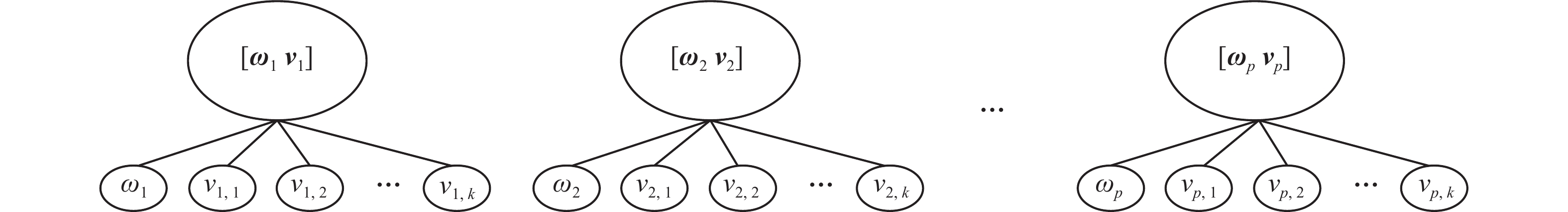图 1 树结构的SGL Fig.1 SGL can be represented as tree structures

1) for i = 1: p do

2) ${\mathit{\boldsymbol{\theta }}_i} = \mathit{\boldsymbol{\theta }}_i^{t + \frac{1}{2}}$

3) for j = 1: k+1

4) if ( $\left| {{\mathit{\boldsymbol{\theta }}_i}[j]} \right| \leqslant {\lambda _2}^\prime$ ) then ${\mathit{\boldsymbol{\theta }}_i}[j] = 0$

5) else $\displaystyle{\mathit{\boldsymbol{\theta }}_i}[j] = \left( {\left. {\frac{{|{\mathit{\boldsymbol{\theta }}_i}[j]| - {\lambda _2}^\prime }}{{|{\mathit{\boldsymbol{\theta }}_i}[j]|}}} \right)} \right. \cdot {\mathit{\boldsymbol{\theta }}_i}[j]$

6) end if

7) end for

8) if ${\left\| {{\mathit{\boldsymbol{\theta }}_i}} \right\|_2} \leqslant {\lambda _1}^\prime$ then ${\mathit{\boldsymbol{\theta }}_i} = 0$

9) else $\displaystyle{\mathit{\boldsymbol{\theta }}_i} = \left( {\frac{{||{\mathit{\boldsymbol{\theta }}_i}|{|_2} - {\lambda _1}^\prime }}{{||{\mathit{\boldsymbol{\theta }}_i}|{|_2}}}} \right) \cdot {\mathit{\boldsymbol{\theta }}_i}$

10) end if

11) end for

1) for k=1:num_epoch %迭代次数

2) 随机排列所有训练样本

3) for i = 1:num_samples %遍历所有样本

4) 取出样本xi

5) 根据式(12)执行随机梯度下降

6) 根据算法1优化式(13)

7) end for

8) end for

3 实验与分析 3.1 实验设置与实验数据表 1 实验数据 Tab.1 Experimental datasets

 $\normalsize{\rm{RMSE}} = \sqrt {\frac{1}{n}\sum\limits_{i = 1}^n {{{({{\hat y}_i} - {y_i})}^2}} }$

 ${\rm{sparsity}} = \frac{{{n_z}}}{{{n_a}}}$

3.2 性能与稀疏度分析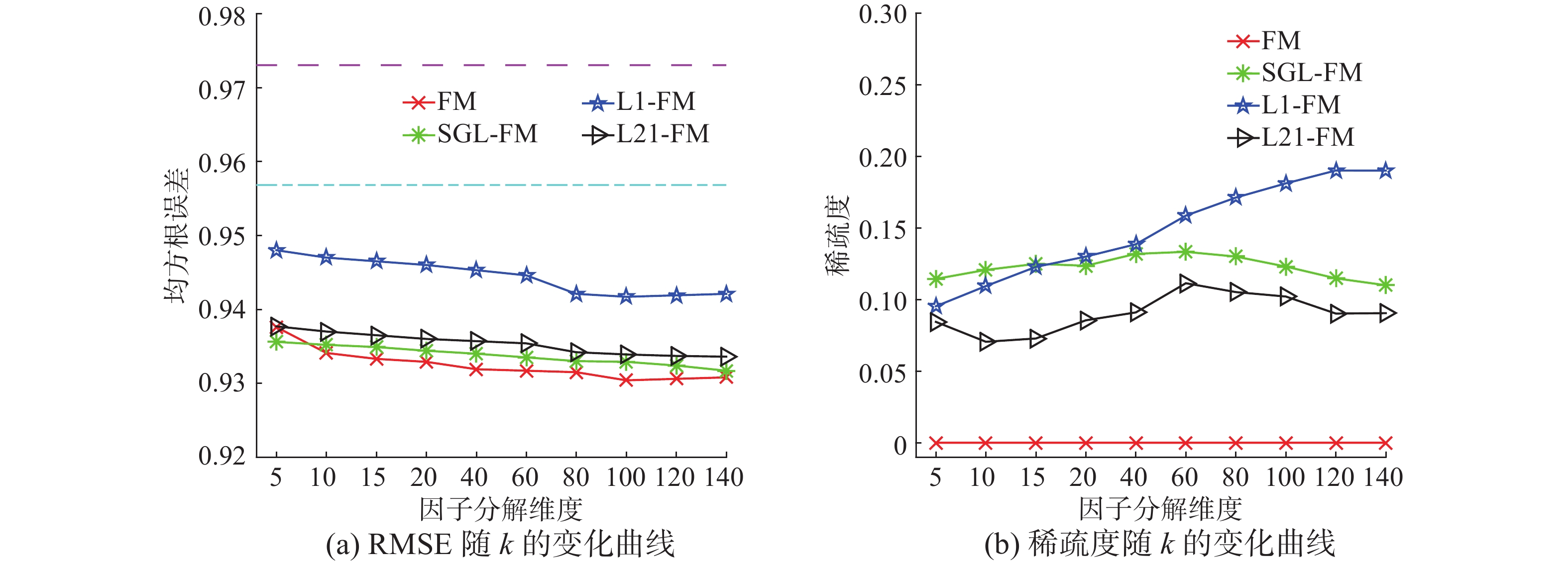图 2 Movielens 100 k实验结果 Fig.2 Results on movielens 100 k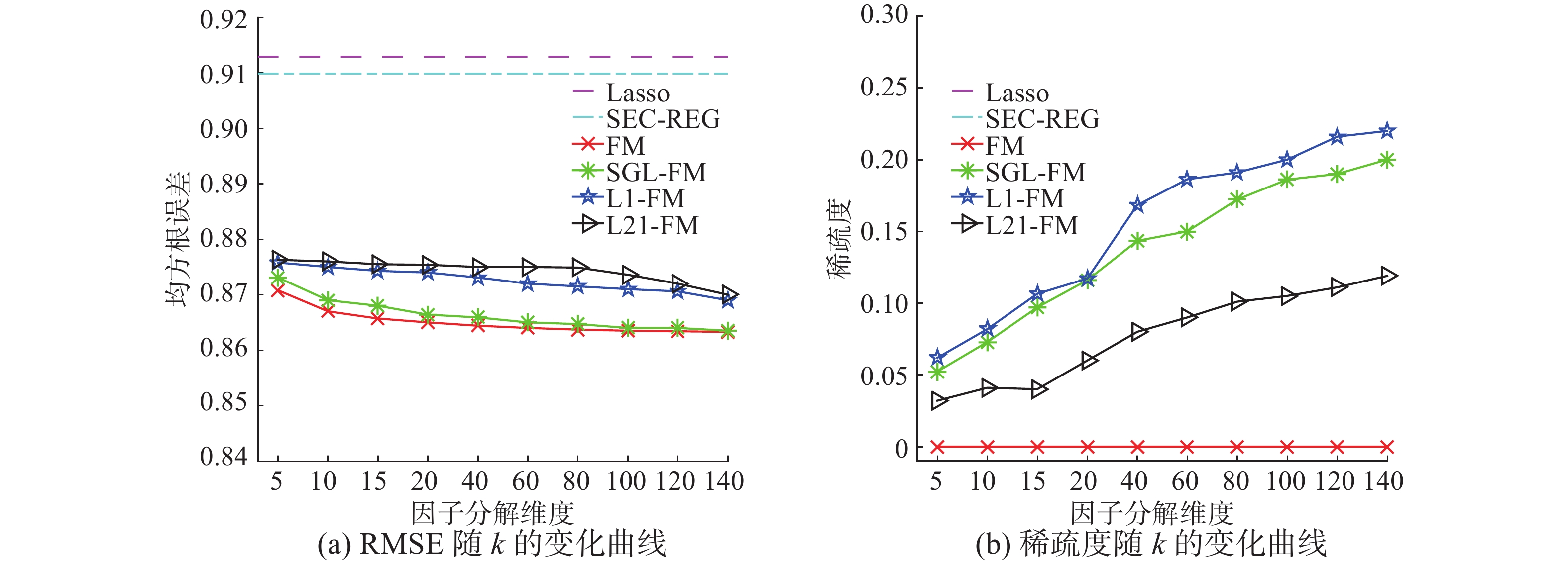图 3 Movielens 1 m实验结果 Fig.3 Fig 2. results on movielens 1 m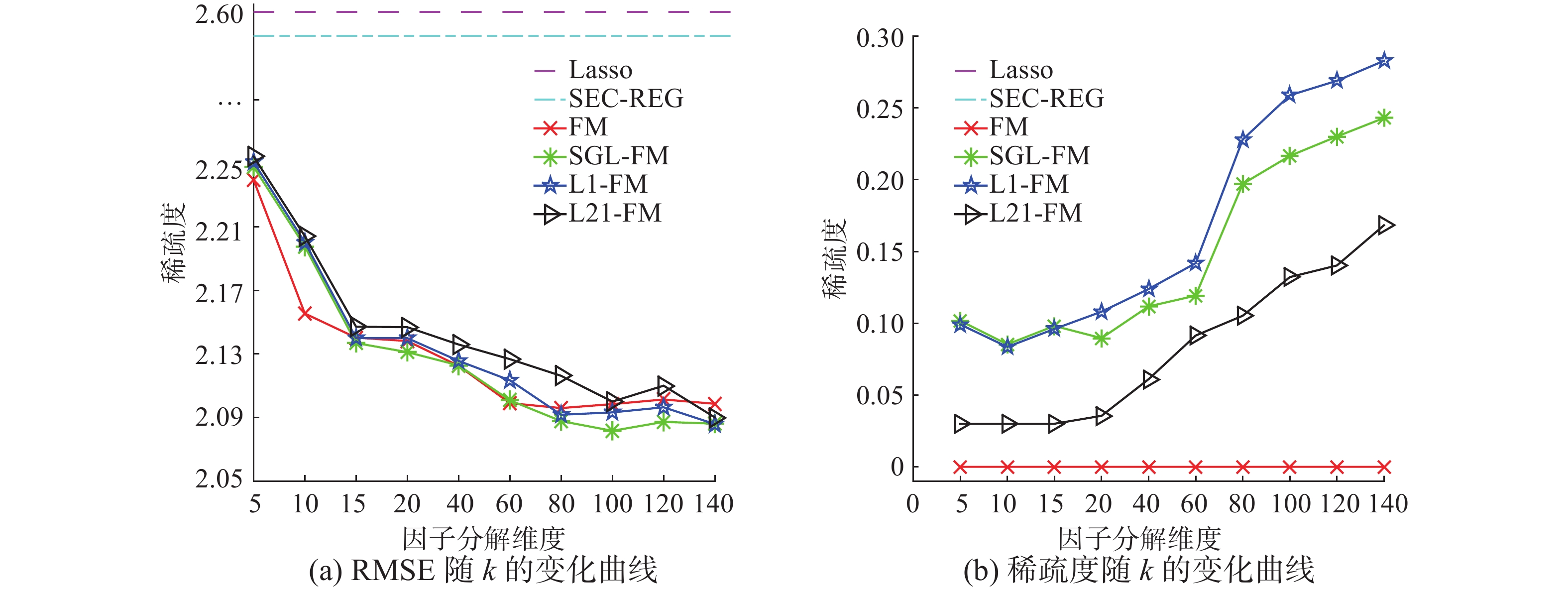图 4 Last.fm实验结果 Fig.4 Results on last.fm图 5 SGL-FM在Last.fm上自适应的k值分布 Fig.5 The distribution of k selected by SGL-FM
3.3 收敛性分析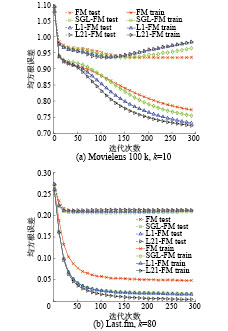图 6 各算法训练和测试RMSE随着迭代次数的变化 Fig.6 The training RMSE and testing RMSE w.r.t the iteration times
4 结束语

  RAO C R, TOUTENBURG H. Linear models[M]. New York: Springer, 1995: 3–18. (0)  ADOMAVICIUS G, TUZHILIN A. Context-aware recommender systems[M]. US: Springer, 2015: 191–226. (0)  RENDLE S. Factorization machines[C]//IEEE 10th International Conference on Data Mining. Sydney, Australia, 2010: 995–1000. (0)  RENDLE S. Learning recommender systems with adaptive regularization[C]//Proceedings of the fifth ACM international conference on Web search and data mining. Seattle, USA, 2012: 133–142. (0)  TIBSHIRANI R. Regression shrinkage and selection via the lasso[J]. Journal of the royal statistical society, Series B (Methodological), 1996, 73(3): 267-288. (0)  YUAN M, LIN Y. Model selection and estimation in regression with grouped variables[J]. Journal of the Royal Statistical Society: Series B (Statistical Methodology), 2006, 68(1): 49-67. (0)  SIMON N, FRIEDMAN J, HASTIE T, et al. A sparse-group lasso[J]. Journal of computational and graphical statistics, 2013, 22(2): 231-245. (0)  BLONDEL M, FUJINO A, UEDA N, et al. Higher-order factorization machines[C]//Advances in Neural Information Processing Systems. Barcelona, Spain 2016: 3351–3359. (0)  LI M, LIU Z, SMOLA A J, et al. DiFacto: distributed factorization machines[C]//Proceedings of the Ninth ACM International Conference on Web Search and Data Mining. San Francisco, USA, 2016: 377–386. (0)  CHIN W S, YUAN B, YANG M Y, et al. An efficient alternating newton method for learning factorization machines [R].NTU:NTU,2016. (0)  DUCHI J, SINGER Y. Efficient online and batch learning using forward backward splitting[J]. Journal of Machine Learning Research, 2009, 10(12): 2899-2934. (0)  RENDLE S. Factorization machines with libfm[J]. ACM transactions on intelligent systems and technolog, 2012, 3(3): 57. (0)  LIU J, YE J. Moreau-Yosida regularization for grouped tree structure learning[C]//Advances in Neural Information Processing Systems. Vancouver, Canada, 2010: 1459–1467. (0)